# Example No. D4(b) Optional Calculation for Multifamily Dwelling

A multifamily dwelling equipped with electric cooking and space heating or air conditioning and having 40 dwelling units.

Meters in two banks of 20 each plus house metering and individual subfeeders to each dwelling unit.

Each dwelling unit is equipped with an electric range of 8-kW nameplate rating, four 1.5-kW separately controlled 240-volt electric space heaters, and a 2.5-kW 240-volt electric water heater. Assume range, space heater, and water heater kW ratings equivalent to kVA.

A common laundry facility is available to all tenants [see 210.52(F), Exception No. 1].

Area of each dwelling unit is 840 square feet.

Calculated Load for Each Dwelling Unit (See Article 220)

 General lighting load: 840 sq. ft. at 3 volt-amperes per sq. ft. 2520 VA Electric range 8000 VA Electric heat, 6 kVA 6000 VA (or air conditioning if larger) ________ Electric water heater 2500 VA

Minimum Number of Branch Circuits Required for Each Dwelling Unit

General lighting: 2520 volt-amperes ÷ 120 volts = 21 amperes. Two 15-ampere 2-wire circuits or two 20-ampere 2-wire circuits.

Small appliance load: Two 2-wire circuits of 12 AWG [see 220.11(C)(1)].

Range circuit (See Table 220.55, column B): 8000 volt-amperes x 80% ÷ 240 volts = 27 amperes on a circuit of three 10 AWG conductors as per 220.19(A)(3).

Space heating: 6000 volt-amperes ÷ 240 volts = 25 amperes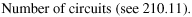Minimum Size Feeder Required for Each Dwelling Unit (See 215.2)

 Calculated load (see Article 220) General lighting load 2520 VA Small appliance load, two 20-ampere circuits 3000 VA Subtotal calculated load (without range and space heating) 5520 VA

Application of Demand Factor

 First 3000 volt-amperes at 100% 3000 VA 5520 VA - 3000 VA = 2520 volt-amperes at 35% 882 VA Net calculated load (without range and space heating) 3882 VA Range 6400 VA Space heating (see 220.51) 6000 VA Water heater 2500 VA Net calculated load (for individual dwelling unit) 18,782 VA

Size of Each Feeder

For 120/240 volt, 3-wire system: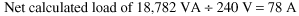Feeder Neutral (See 220.61)

 Lighting and small appliance load 3882 VA Range 6400 at 70% [see 220.61(B)] 4480 VA Space and water heating (no neutral) 240 volt 0 VA Net calculated load (neutral) 8362 VA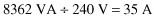Minimum Size Feeder Required from Service Equipment to Meter Bank (for 20 Dwelling Units)

 Total calculated load: Lighting and small appliance load, 20 units x 5520 volt-amperes 110,400 VA Water and space heating load, 20 units x 8500 volt-amperes 170,000 VA Range load, 20 x 8000 160,000 VA Net calculated load (20 dwelling units) 440,400 VA Net calculated load using optional calculation (see Table 220.84) 440,400 volt-amperes x 0.38 167,352 VAMinimum Size Main Feeder Required (Less House Load) (for 40 Dwelling Units)

 Calculated load Lighting and small appliance load, 40 units x 5520 volt-amperes 220,800 VA Water and space heating, 40 units x 8500 volt-amperes 340,000 VA Range: 40 ranges x 8000 volt-amperes 320,000 VA Net calculated load (40 dwelling units) 880,800 VA Net calculated load using optional calculation (See Table 220.84) 880,800 volt-amperes x 0.28 = 246,624 VA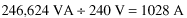Feeder Neutral Load for Feeder from Service Equipment to Meter Bank (for 20 Dwelling Units)

 Lighting and small appliance load, 20 units x 5520 volt-amperes 110,400 VA First 3000 volt-amperes at 100% 3,000 VA 110,000 VA - 3000 VA = 107,400 volt-amperes at 35% 37,590 VA Net calculated load 40,590 VA 20 ranges: 35,000 volt-amperes at 70% [see Table 220.55 and 220.61(B)] 24,500 VA Total 65,090 VA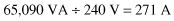Further Demand Factor [See 220.61(B)]

 First 200 amperes at 100% 200 A Balance: 271 A - 200 A = 71 amperes at 70% 50 A Total 250 A

Feeder Neutral Load of Mains Feeder (Less House Load) (for 40 Dwelling Units)

 Lighting and small appliance load, 40 x 5520 volt-amperes 220,800 VA First 3000 volt-amperes at 100% 3,000 VA Next 120,000 volt-amperes - 3000 volt- amperes = 117,000 volt-amperes at 35% 40,950 VA Remainder 220,800 volt-amperes - 120,000 volt-amperes = 100,800 volt-amperes at 25% 25,200 VA Net calculated load 69,150 VA 40 ranges: 55,000 volt-amperes at 70% [see Table 220.55 and 220.61(B)] 38,500 VA Total 107,650 VAFurther demand factor [see 220.61(B)]

 First 200 amperes at 100% 200 A Balance: 449 A - 200 A = 249 amperes at 70% 174 A Total 374 A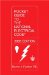Pocket Guide to the National Electrical Code(R), 2005 Edition (8th Edition)
ISBN: 0131480014
EAN: 2147483647
Year: 2004
Pages: 120